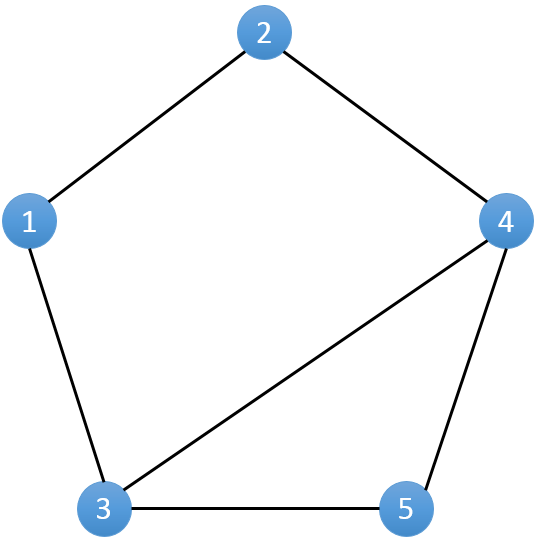# Bipartial Graph - Part I - 二分图一•二分图判定

## Question

### Problem Statement

#### 描述

OK，让我们愉快的暴力搜索吧！1. 选取一个未染色的点u进行染色
2. 遍历u的相邻节点v：若v未染色，则染色成与u不同的颜色，并对v重复第2步；若v已经染色，如果 u和v颜色相同，判定不可行退出遍历。
3. 若所有节点均已染色，则判定可行。

#### 输出

2
5 5
1 2
1 3
3 4
5 2
1 5
5 5
1 2
1 3
3 4
5 2
3 5


Wrong
Correct


## 题解

### Java

import java.util.*;
import java.util.Queue;

class UndirectedGraphNode {
int label;
int color;
ArrayList<UndirectedGraphNode> neighbors;
UndirectedGraphNode(int x) {
this.label = x;
this.color = 0;
this.neighbors = new ArrayList<UndirectedGraphNode>();
}
}

public class Main {
public static void main(String[] args) {
Scanner in = new Scanner(System.in);
int T = in.nextInt();
for (int i = 1; i <= T; i++) {
int N = in.nextInt();
int M = in.nextInt();
// initialize graph
List<UndirectedGraphNode> graph = new ArrayList<UndirectedGraphNode>();
for (int n = 1; n <= N; n++) {
}
// construct graph
for (int j = 1; j <= M; j++) {
int u = in.nextInt(), v = in.nextInt();
}
// solve
if (solve(graph)) {
System.out.println("Correct");
} else {
System.out.println("Wrong");
}
}
}

public static boolean solve(List<UndirectedGraphNode> graph) {
// 1 for white, -1 for black, 0 for uncolored
for (UndirectedGraphNode node : graph) {
if (node.color == 0) {
node.color = 1;
q.offer(node);
while (!q.isEmpty()) {
int qSize = q.size();
for (int i = 0; i < qSize; i++) {
UndirectedGraphNode qNode = q.poll();
for (UndirectedGraphNode neighbor : qNode.neighbors) {
if (neighbor.color == 0) {
neighbor.color = -1 * qNode.color;
q.offer(neighbor);
} else if (neighbor.color + qNode.color != 0) {
// the color of qNode is the same with neighbor
return false;
}
}
}
}
}
}

return true;
}
}